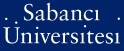# Equilibrium constrained optimization problems

Birbil, Ş. İlker and Bouza, G. and Frenk, J. B. G. and Still, G. (2006) Equilibrium constrained optimization problems. European Journal of Operational Research, 169 (3). pp. 1108-1127. ISSN 0377-2217PDF - Registered users only - Requires a PDF viewer such as GSview, Xpdf or Adobe Acrobat Reader262Kb

Official URL: http://dx.doi.org/10.1016/j.ejor.2004.07.075

## Abstract

We consider equilibrium constrained optimization problems, which have a general formulation that encompasses well-known models such as mathematical programs with equilibrium constraints, bilevel programs, and generalized semi-infinite programming problems. Based on the celebrated KKM lemma, we prove the existence of feasible points for the equilibrium constraints. Moreover, we analyze the topological and analytical structure of the feasible set. Alternative formulations of an equilibrium constrained optimization problem (ECOP) that are suitable for numerical purposes are also given. As an important first step for developing efficient algorithms, we provide a genericity analysis for the feasible set of a particular ECOP, for which all the functions are assumed to be linear.

Item Type: Article equilibrium problems; existence of feasible points; mathematical programs with equilibrium constraints; problems with complementarity constraints; bilevel programs; generalized semi-infinite programming; genericity Q Science > QA Mathematics 654 Ş. İlker Birbil 19 Dec 2006 02:00 25 May 2011 14:03

Repository Staff Only: item control page